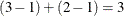# The TRANSREG Procedure

### Main-Effects ANOVA

This example shows how to use PROC TRANSREG to code and fit a main-effects ANOVA model. PROC TRANSREG has very extensive and versatile options for coding or creating so-called dummy variables. PROC TRANSREG is commonly used to code classification variables before they are used for analysis in other procedures. See the sections Using the DESIGN Output Option and Discrete Choice Experiments: DESIGN, NORESTORE, NOZERO. In this example, the input data set contains the dependent variables `y`, factors `x1` and `x2`, and 12 observations. PROC TRANSREG can be useful for coding even before running procedures with a CLASS statement because of its detailed options that enable you to control how the coded variable names and labels are constructed. The following statements perform a main-effects ANOVA and display the results in Figure 104.12 and Figure 104.13:

```title 'Introductory Main-Effects ANOVA Example';

data a;
input y x1 \$ x2 \$;
datalines;
8 a a
7 a a
4 a b
3 a b
5 b a
4 b a
2 b b
1 b b
8 c a
7 c a
5 c b
2 c b
;
```
```* Fit a main-effects ANOVA model with 1, 0, -1 coding;
proc transreg ss2;
model identity(y) = class(x1 x2 / effects);
output coefficients replace;
run;

* Display TRANSREG output data set;
proc print label;
format intercept -- x2a 5.2;
run;
```

The SS2 a-option requests results based on Type II sums of squares. The simple ANOVA model is fit by designating `y` as an IDENTITY variable, which specifies no transformation. The independent variables are specified with a CLASS expansion, which replaces them with coded variables. There arecoded variables created by the CLASS specification, since the two CLASS variables have 3 and 2 different values or levels. In this case, the EFFECTS t-option is specified. This option requests an effects coding (displayed in Figure 104.13), which is also called a deviations from means or 0, 1, –1 coding. The OUTPUT statement requests an output data set with the data and coded variables. The COEFFICIENTS output option, or o-option, adds the parameter estimates and marginal means to the data set. The REPLACE o-option specifies that the transformed variables should replace the original variables in the output data set. The output data set variable names are the same as the original variable name. In an example like this, there are no nonlinear transformations; the transformed variables are the same as the original variables. The REPLACE o-option is used to eliminate unnecessary and redundant transformed variables from the output data set. The results of the PROC TRANSREG step are shown in Figure 104.12.

Figure 104.12: ANOVA Example Output from PROC TRANSREG

 Introductory Main-Effects ANOVA Example

The TRANSREG Procedure

 Dependent Variable Identity(y)

Class Level Information
Class Levels Values
x1 3 a b c
x2 2 a b

 Number of Observations Read 12 12

 The TRANSREG Procedure Hypothesis Tests for Identity(y)

Univariate ANOVA Table Based on the Usual Degrees of Freedom
Source DF Sum of Squares Mean Square F Value Pr > F
Model 3 57.00000 19.00000 19.83 0.0005
Error 8 7.66667 0.95833
Corrected Total 11 64.66667

 Root MSE R-Square 0.97895 0.8814 4.66667 0.8370 20.9774

Univariate Regression Table Based on the Usual Degrees of Freedom
Variable DF Coefficient Type II
Sum of
Squares
Mean Square F Value Pr > F Label
Intercept 1 4.6666667 261.333 261.333 272.70 <.0001 Intercept
Class.x1a 1 0.8333333 4.167 4.167 4.35 0.0705 x1 a
Class.x1b 1 -1.6666667 16.667 16.667 17.39 0.0031 x1 b
Class.x2a 1 1.8333333 40.333 40.333 42.09 0.0002 x2 a

Figure 104.12 shows the ANOVA results, fit statistics, and regression tables. The output data set, with the coded design, parameter estimates and means, is shown in Figure 104.13. For more information about PROC TRANSREG for ANOVA and other codings, see the section ANOVA Codings.

Figure 104.13: Output Data Set from PROC TRANSREG

 Introductory Main-Effects ANOVA Example

Obs _TYPE_ _NAME_ y Intercept x1 a x1 b x2 a x1 x2
1 SCORE ROW1 8 1.00 1.00 0.00 1.00 a a
2 SCORE ROW2 7 1.00 1.00 0.00 1.00 a a
3 SCORE ROW3 4 1.00 1.00 0.00 -1.00 a b
4 SCORE ROW4 3 1.00 1.00 0.00 -1.00 a b
5 SCORE ROW5 5 1.00 0.00 1.00 1.00 b a
6 SCORE ROW6 4 1.00 0.00 1.00 1.00 b a
7 SCORE ROW7 2 1.00 0.00 1.00 -1.00 b b
8 SCORE ROW8 1 1.00 0.00 1.00 -1.00 b b
9 SCORE ROW9 8 1.00 -1.00 -1.00 1.00 c a
10 SCORE ROW10 7 1.00 -1.00 -1.00 1.00 c a
11 SCORE ROW11 5 1.00 -1.00 -1.00 -1.00 c b
12 SCORE ROW12 2 1.00 -1.00 -1.00 -1.00 c b
13 M COEFFI y . 4.67 0.83 -1.67 1.83
14 MEAN y . . 5.50 3.00 6.50

The output data set has three kinds of observations, identified by values of `_TYPE_` as follows:

• When `_TYPE_`=’SCORE’, the observation contains the following information about the dependent and independent variables:

• `y` is the original dependent variable.

• `x1` and `x2` are the independent classification variables, and the `Intercept` through `x2 a` columns contain the main-effects design matrix that PROC TRANSREG creates. The variable names are `Intercept`, `x1a`, `x1b`, and `x2a`. Their labels are shown in the listing.

• When `_TYPE_`=’M COEFFI’, the observation contains coefficients of the final linear model (parameter estimates).

• When `_TYPE_`=’MEAN’, the observation contains the marginal means.

The observations with `_TYPE_`=’SCORE’ form the score or data partition of the output data set, and the observations with `_TYPE_`=’M COEFFI’ and `_TYPE_`=’MEAN’ form the output statistics partition of the output data set.